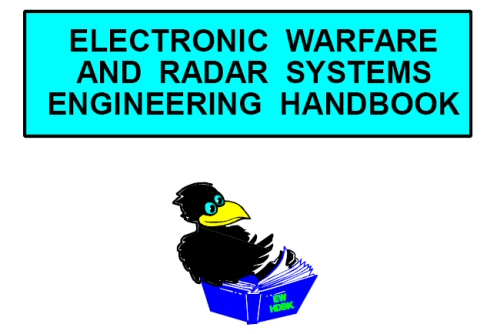# Corner Reflectors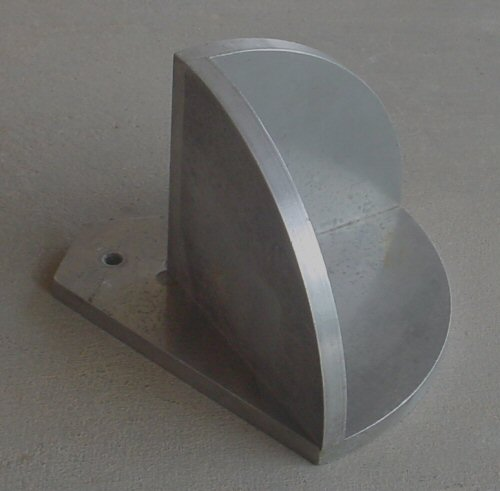A proposed Microwaves101 rule of thumb: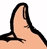The side dimension of a cube corner reflector is ideally greater than 10 wavelengths of the signal you are trying to reflect. Any comments are appreciated!

What's a corner reflector? It's a structure that is used as a radar target, often in calibrating test equipment such as in an anechoic chamber. Corner reflectors are used for many reasons: they have very high radar-cross-section (RSC) for a small size, the high RCS is maintained over a wide incidence angle, and an exact solution is known for their RCS. Corner reflectors are easy to make from sheet metal such as aluminum, but care must be used to be sure that the surfaces join at exactly at 90 degrees, and they are robust enough to maintain good flatness after your "range boy" comes back from a martini lunch and drops them on a hard floor a couple of times!

There are two main types of corner reflectors, dihedral and trihedral. The dihedral has two surfaces that are on orthogonal planes, the trihedral has three. Sketches of the two are shown below, along with generally used coordinate systems. The conventions of using phi for elevation and theta for azimuth angle are used here, as in most antenna work. Note that the spherical coordinate systems are such that angles of zero for both azimuth and elevation give the maximum, (often called specular) return.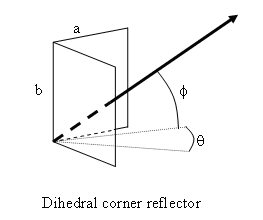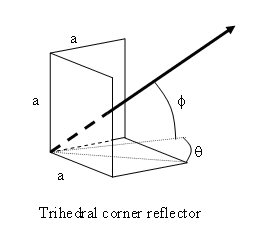Where might you encounter a corner reflector in "real life?" Notice that red taillights on vehicles "light up" when they are illuminated by your headlamps, even when they are on parked cars that are switched off. This is because they are constructed to have hundreds or even thousands of tiny optical corner reflectors built in. Optical corner reflectors work exactly as RF corner reflectors, even if the materials and dimensions are slightly different.

For the equations on this page we reference "Radar Cross Section" by Knott, Shaeffer and Tuley. Lower-case Greek letter sigma (σ) is used to denote radar cross section. Here's the equations for maximum cross-section of both types of corner reflector, along their principal axes:

Dihedral: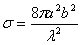Trihedral: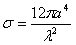Note that for the trihedral case, the formula is accurate for reflectors with square sides. If you cut them into triangles or arcs (like the photo at the top of the page), the constant "12" will be reduced (sorry, we don't have those exact expressions).

While we're at it let's provide the equation for a flat-plate reflector's specular return: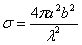Notice the RCS goes up as the fourth power of the side dimension "a" for trihedral. If you double the lengths of the edges, the RCS goes up by a factor of 16. Yikes! The trihedral corner reflector has the strongest return for its size of any object.

When we are discussing RCS here, we mean the maximum RCS at the most favorable angle, which in the case of a corner reflector is 35.3 degrees from each plane surface. The trihedral corner reflector has a good return over a wide look angle, perhaps a 10 degree displacement you won't even notice a reduction in returned signal. Many times in practice the trihedral is used as a dihedral, because of the convenience of just setting it on a horizontal surface in the range. The cross-section is only reduced about -1.8 dB (in this case 10xlog(2/3) is the exact solution).

The RCS is also a function of the frequency squared. It is customary to write the equation using the wavelength in the denominator. Be sure to use the same units for "a" as you do for lambda (λ).

Below we have plotted the RCS for a trihedral corner reflector, with side dimension 10, 15 and 20 centimeters. At X-band the RCS of the 20 cm reflector is already almost 100 square meters.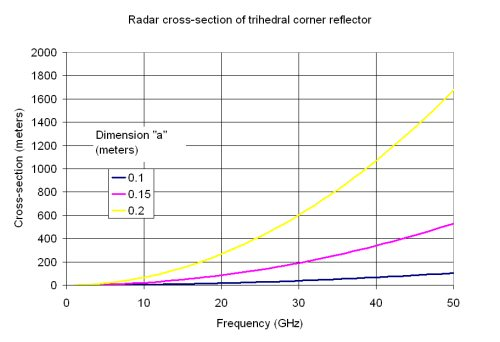### Further information on RCS and corner reflectors

The Navy's publicly-released Electronic Warfare and Radar Systems Engineering Handbook has information on RCS, we have it here: